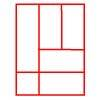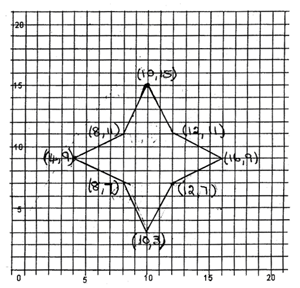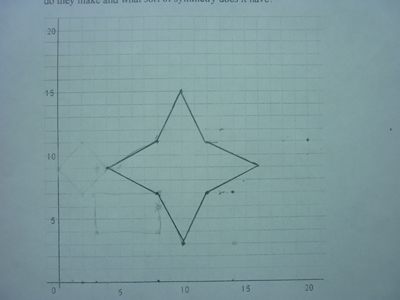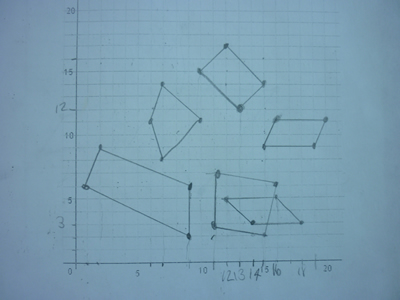#### You may also like### Bracelets

Investigate the different shaped bracelets you could make from 18 different spherical beads. How do they compare if you use 24 beads?### Cut and Make

Cut a square of paper into three pieces as shown. Now,can you use the 3 pieces to make a large triangle, a parallelogram and the square again?### Is a Square a Rectangle?

How many rectangles can you find in this shape? Which ones are differently sized and which are 'similar'?

# A Cartesian Puzzle

##### Age 7 to 11Challenge Level

We have had the following solution from Matthew, David and Jack at St. Nicolas School, Newbury. They thought there was a problem with number 6 but there isn't!

Our solutions to the missing coordinates are:

1. (2,11), (0,9), (2,7) ..............(4,9)
which had both rotational and line symmetry.
2. (3,7), (3,4), (8,4) ..............(8,7)
which had both rotational and line symmetry.
3. (18,3), (16,5), (12,5) ..............(10,3)
4. (13,12), (15,14), (12,17) ..............(10,15)
which had both rotational and line symmetry.
5. (7,14), (6,11), (7,8) ..............(8,11)
which had both rotational and line symmetry.
6. (15,9), (19,9), (16,11) ..............(12,11)
7. (11,3), (15,2), (16,6) ..............(12,7)
which had both rotational and line symmetry.
8. (9,16), (2,9), (9,2) ..............(16,9)
which had both rotational and line symmetry.

We plotted these 8 sets of coordinates, which made a symmetrical star.We also heard from George and Thalia from Hoyle Court Primary School, Baildon who worked together to complete the puzzle.  Their teacher wrote:

They worked out the missing co-ordinates for the quadrilaterals then tried to produce the final symmetrical shape.
At first their shape was not symmetrical. They realised it should be a star and corrected the two inaccurate co-ordinates.
Then went back and drew the original two quadrilaterals again using the amended co-ordinates from the star.
Great teamwork!!

Here are photos of their symmetrical star and some of their initial quadrilaterals:The students in 5D at Vienna International School have been working on  this.
They think they have come up with a way to find the missing coordinate without using the grid.
Let's take the first example: (2,11), (0,9), (2,7)

Take the first and third x axis coordinate and add them together. Subtract the second x axis coordinate. This give you 4.
Take the first and third y axis coordinate and add them together. Subtract the second y axis coordinate. This give you 9..

Their process seems to work for all of the examples here. They drew their own symmetrical quadrilaterals and gave three coordinates in an anticlockwise direction to a friend. Using the same process their friends tried to work out the fourth coordinate. It worked in each case.

Well done to Chrysanthi, Kathy, Jack, Samuel, Erik, Timothy for their persistence with this.